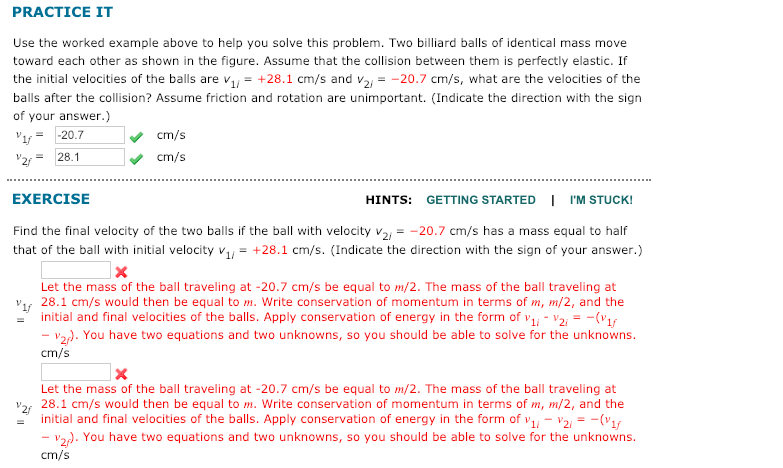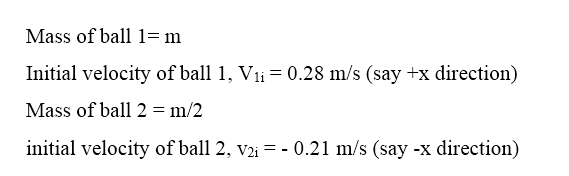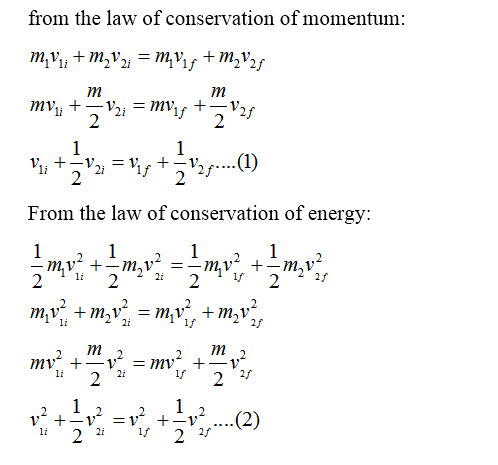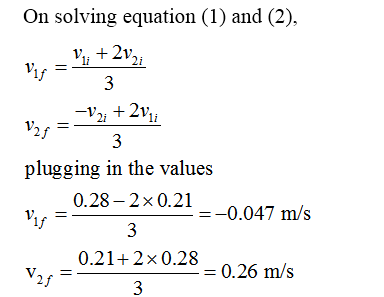# PRACTICE ITUse the worked example above to help you solve this problem. Two billiard balls of identical mass movetoward each other as shown in the figure. Assume that the collision between them is perfectly elastic. Ifthe initial velocities of the balls are v = +28.1 cm/s and v2 = -20.7 cm/s, what are the velocities of theballs after the collision? Assume friction and rotation are unimportant. (Indicate the direction with the signofyour answer.)-20.7cm/s28.1cm/sEXERCISEHINTS: GETTING STARTED I I'M STUCK!Find the final velocity of the two balls if the ball with velocity v2, = -20.7 cm/s has a mass equal to halfthat of the ball with initial velocity v1/ = +28.1 cm/s. (Indicate the direction with the sign of your answer.)Let the mass of the ball traveling at -20.7 cm/s be equal to m/2. The mass of the ball traveling atvs 28.1 cm/s would then be equal to m. Write conservation of momentum in terms of m, m/2, and theinitial and final velocities of the balls. Apply conservation of energy in the form of v1; - v2 = -(V1- v2). You have two equations and two unknowns, so you should be able to solve for the unknowns.cm/sLet the mass of the ball traveling at -20.7 cm/s be equal to m/2. The mass of the ball traveling atV2, 28.1 cm/s would then be equal to m. Write conservation of momentum in terms of m, m/2, and theinitial and final velocities of the balls. Apply conservation of energy in the form of v1, - V21 = -(V1- v2). You have two equations and two unknowns, so you should be able to solve for the unknowns.cm/s

Question
1 viewshelp_outlineImage TranscriptionclosePRACTICE IT Use the worked example above to help you solve this problem. Two billiard balls of identical mass move toward each other as shown in the figure. Assume that the collision between them is perfectly elastic. If the initial velocities of the balls are v = +28.1 cm/s and v2 = -20.7 cm/s, what are the velocities of the balls after the collision? Assume friction and rotation are unimportant. (Indicate the direction with the sign of your answer.) -20.7 cm/s 28.1 cm/s EXERCISE HINTS: GETTING STARTED I I'M STUCK! Find the final velocity of the two balls if the ball with velocity v2, = -20.7 cm/s has a mass equal to half that of the ball with initial velocity v1/ = +28.1 cm/s. (Indicate the direction with the sign of your answer.) Let the mass of the ball traveling at -20.7 cm/s be equal to m/2. The mass of the ball traveling at vs 28.1 cm/s would then be equal to m. Write conservation of momentum in terms of m, m/2, and the initial and final velocities of the balls. Apply conservation of energy in the form of v1; - v2 = -(V1 - v2). You have two equations and two unknowns, so you should be able to solve for the unknowns. cm/s Let the mass of the ball traveling at -20.7 cm/s be equal to m/2. The mass of the ball traveling at V2, 28.1 cm/s would then be equal to m. Write conservation of momentum in terms of m, m/2, and the initial and final velocities of the balls. Apply conservation of energy in the form of v1, - V21 = -(V1 - v2). You have two equations and two unknowns, so you should be able to solve for the unknowns. cm/s fullscreen
check_circle

Step 1Step 2Step 3...

### Want to see the full answer?

See Solution

#### Want to see this answer and more?

Solutions are written by subject experts who are available 24/7. Questions are typically answered within 1 hour.*

See Solution
*Response times may vary by subject and question.
Tagged in